﻿ Bgods - ggplot2学习笔记之分面

# ggplot2学习笔记之分面

## 1．网格分面（facet_grid）

• 不进行分面：即不使用函数facet_grid()或者使用facet_null()，此时得到的是单独画板；
``````ggplot(mtcars, aes(mpg, wt)) + geom_point()
ggplot(mtcars, aes(mpg, wt)) + geom_point() + facet_null() #等价于以上
``````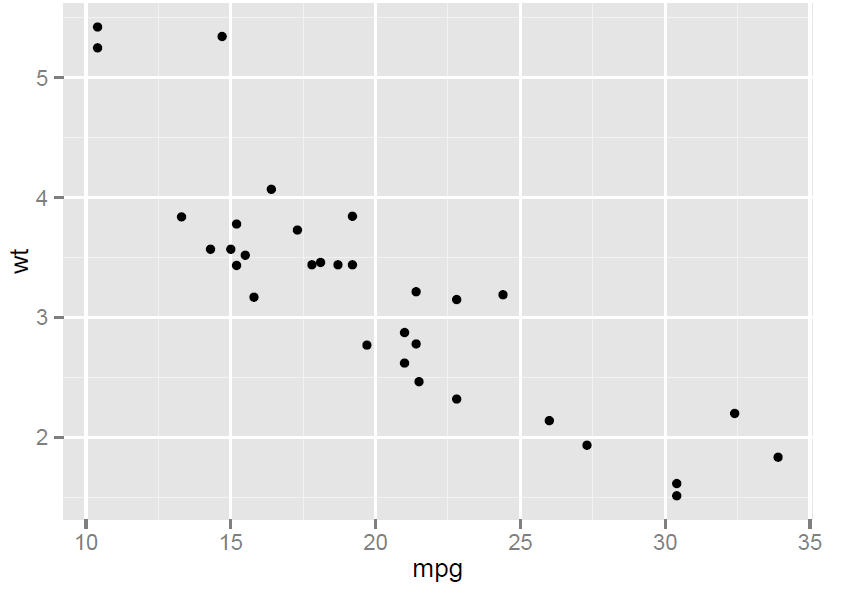• 一行多列：“.\~A”。图形以一行多列展示，纵坐标相同，用于y 位置的比较。
``````ggplot(iris, aes(Sepal.Length, Sepal.Width)) + geom_point() + facet_grid('. ~ Species')
``````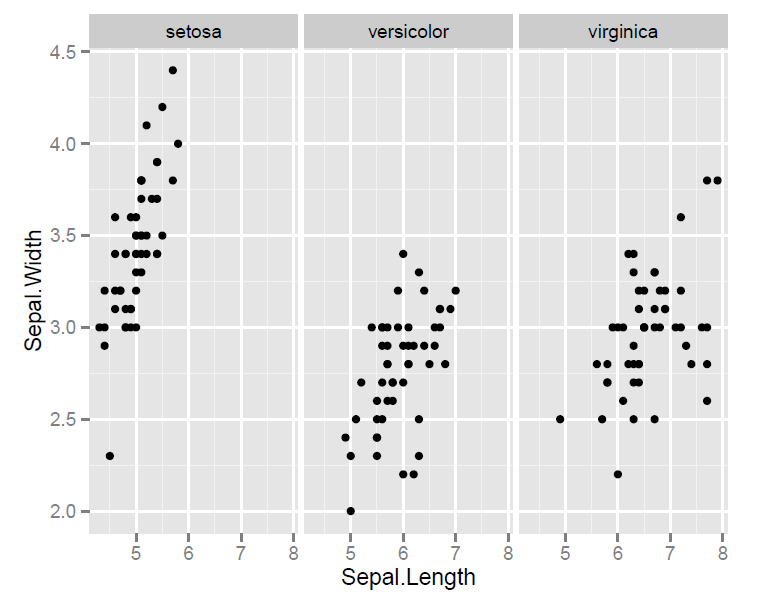Species 变量有三个属性值，按其分组，绘制一行三列的图形，x 轴相同，通过查看y 轴的比较三者的不同。

• 一列多行：“B\~.”。图形以一列多行展示，横坐标相同，用于x 位置的比较。
``````ggplot(iris, aes(Sepal.Length, Sepal.Width)) + geom_point() + facet_grid('Species ~ .')
``````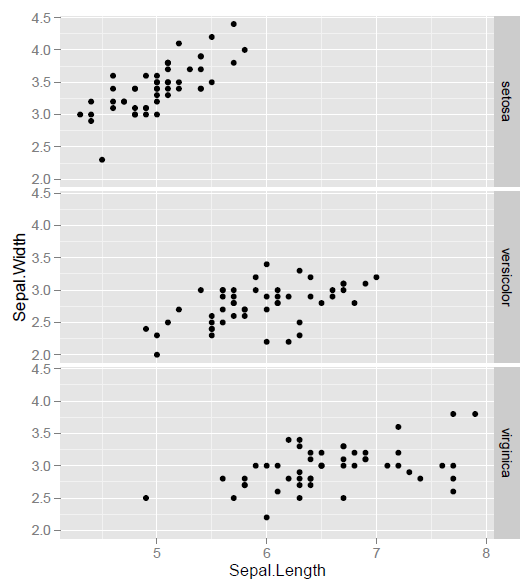• 多列多行：“B\~A”。按照A 与B 变量分组绘图；
``````h <- ggplot(mtcars, aes(x = mpg, y = wt)) + geom_point()
h + facet_grid(cyl ~ vs)
``````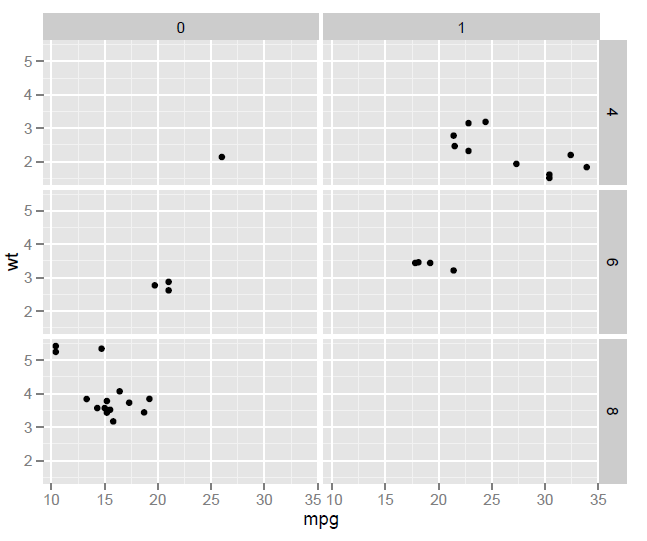• 多个变量的多个水平行或者列上（或者同时）：”A+B\~.”、”.\~A+B”、 ”A+B\~C”。
``````mg <- ggplot(mtcars, aes(x = mpg, y = wt)) + geom_point()
mg + facet_grid(vs + am ~ gear)
``````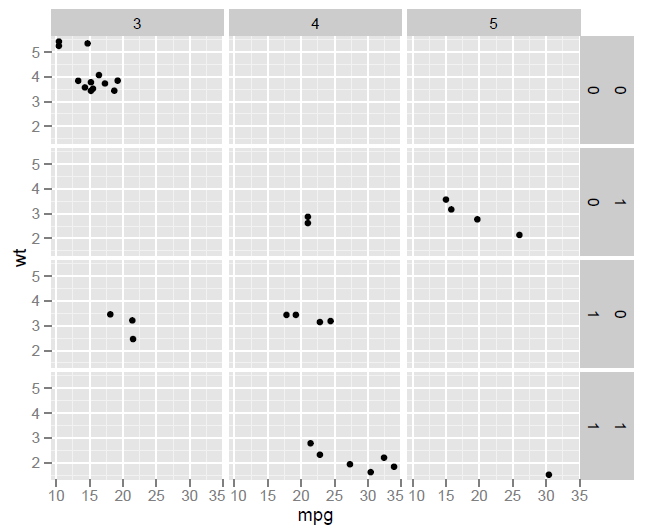``````mg + facet_grid(vs + am ~ gear, margins = TRUE) #参数margins = TRUE 表示展示所有的边际图
``````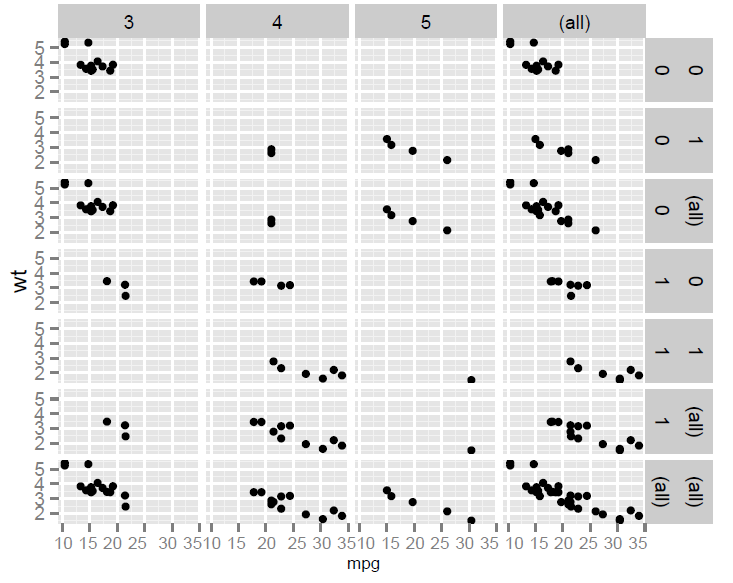``````mg + facet_grid(vs + am ~ gear, margins = "am") #参数margins = "am"表示展示am 边际图
``````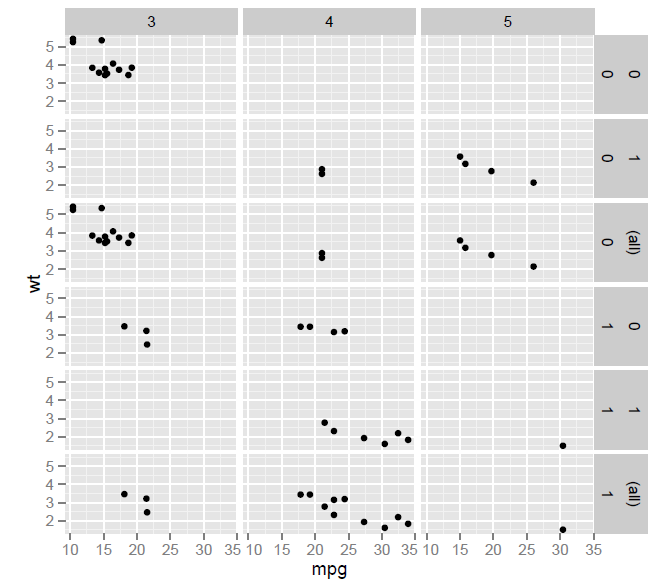## 2．封装型（facet_wrap）

facet_wrap 并不是用于两个或者更多的变量来生成一个2 维网格，而是生成一个长的面板条块（由任意数目的变量生成），然后封装在2 维中。

``````p <- qplot(price, data = diamonds, geom = "histogram", binwidth = 1000)
p + facet_wrap(~ color)
``````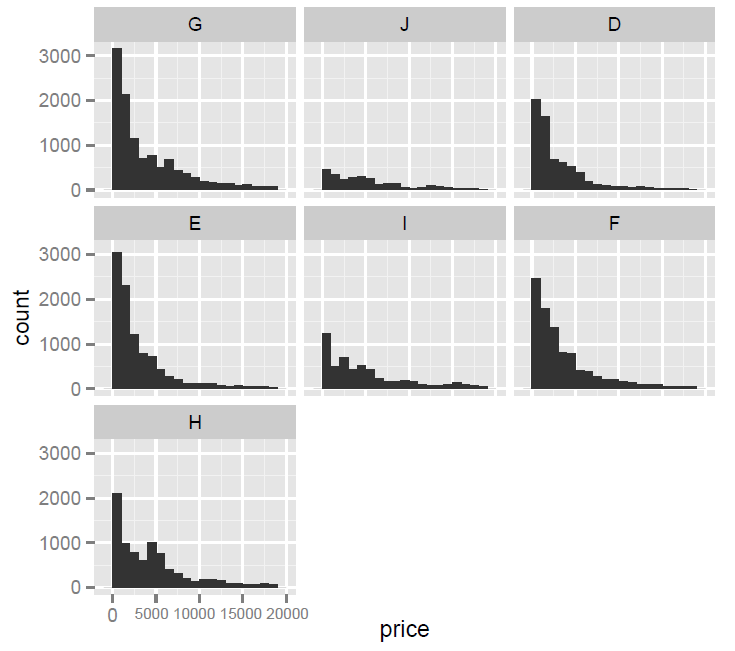``````p + facet_wrap(~ color, nrow = 2) #图形按两行显示，参数ncol 是设置列的
``````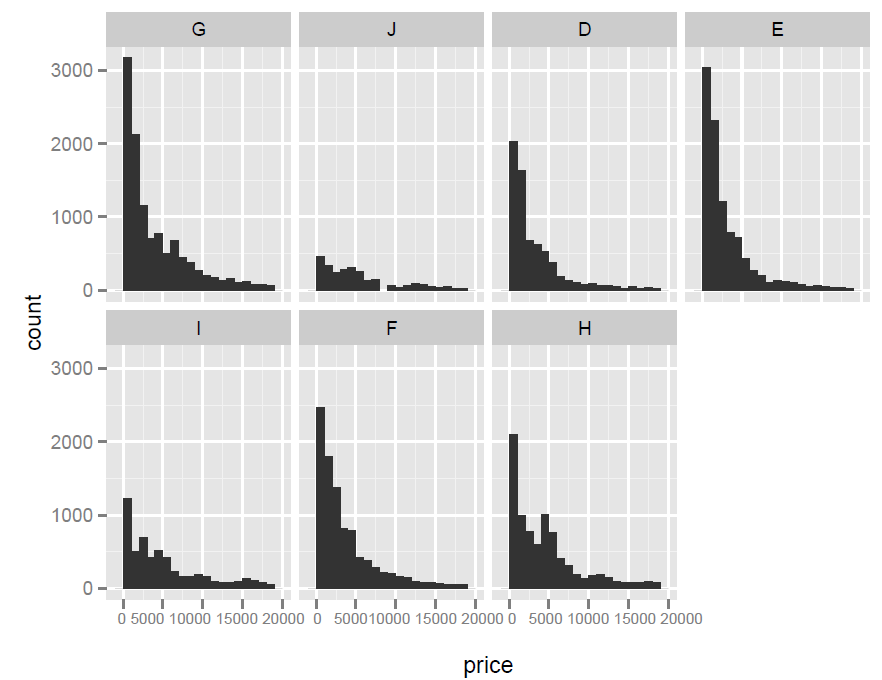``````p + facet_wrap(~ color, scales = "free_x") #所有图形都显示x 轴坐标，y 轴是参数“free_y”
``````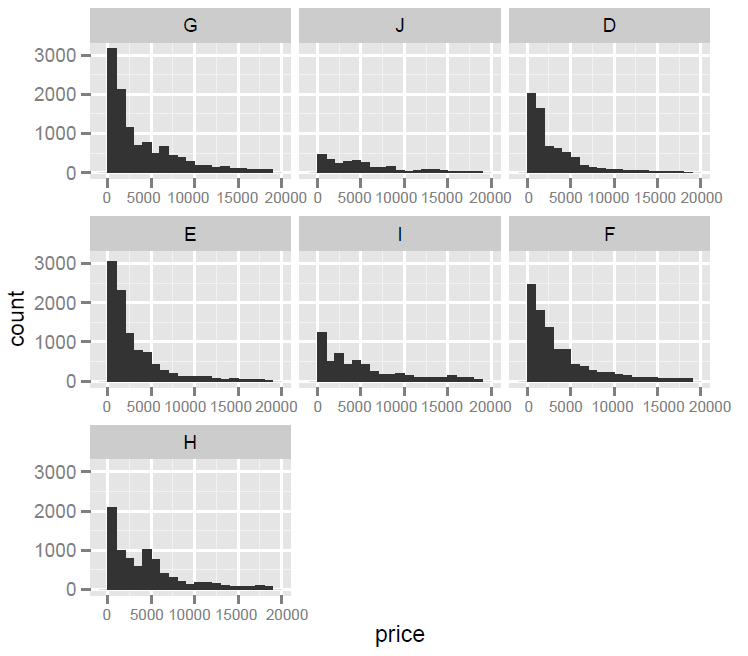``````p + facet_wrap(~ color, scales = "free") #所有图形都显示x、y 轴坐标
``````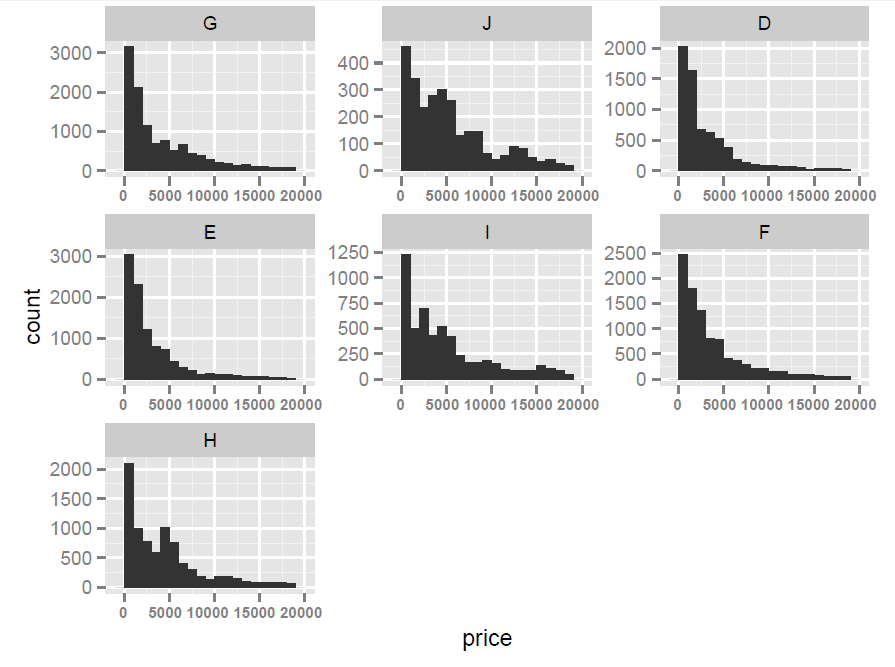0 评论